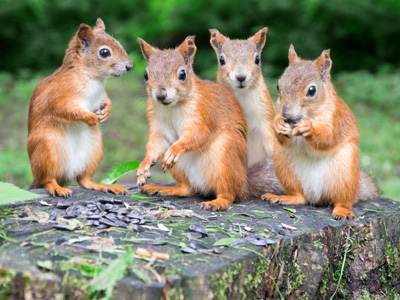How many squirrels? 1 + 3 = 4 in total.

# How Much in Total?

This Math quiz is called 'How Much in Total?' and it has been written by teachers to help you if you are studying the subject at elementary school. Playing educational quizzes is a fun way to learn if you are in the 1st or 2nd grade - aged 6 to 8.

It costs only \$12.50 per month to play this quiz and over 3,500 others that help you with your school work. You can subscribe on the page at Join Us

Understanding and using the words associated with addition is an important first step when calculating. Recognizing that 'finding the total' means finding how much or how many there are altogether, especially when groups of numbers or objects are added, is important as 'total' is one of the first addition words elementary school children will encounter.

Can you find the totals?

Click on the pictures for a closer look.

1.
What is the total number of crayons in this pot?
11
12
10
13
There is a total of 12 crayons
2.
What is the total number of birds?
9
8
10
7
2 + 5 + 2 gives a total of 9
3.
What does 'total' mean?
A subtraction must be done
How many there are altogether
What you get when you divide the numbers
What you get if you count backwards
The total is how many there are altogether if you count or add everything together
4.
How many stars in total?
8
4
6
9
4 + 4 gives a total of 8
5.
What is the total shown on the two dice?
3
4
7
10
4 + 3 gives a total of 7
6.
How many fish are there in total?
15
12
9
10
There is a total of 10 fish. Did you spot them all?
7.
How many dogs in total?
5
6
7
8
There is a total of 5 dogs here
8.
How many socks in total?
6
2
4
8
4 pairs of socks is a total of 8
9.
How many chocolates in total?
12
14
15
16
4 + 4 + 4 + 4 gives a total of 16
10.
How many children in total?
8
9
7
10
There are 10 children in total
Author:  Angela Smith